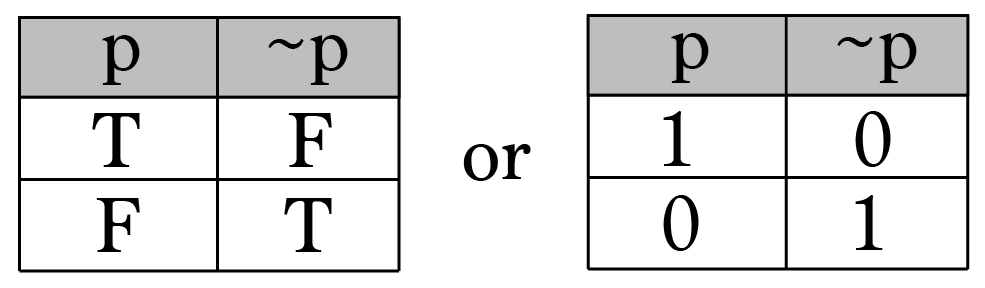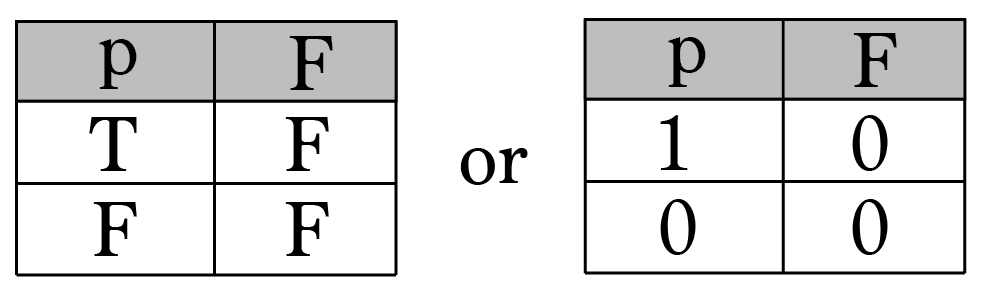# Discrete Math Logical Equivalence

Logical equivalence is a type of relationship between two statements or sentences in propositional logic or Boolean algebra.

You can’t get very far in logic without talking about propositional logic also known as propositional calculus.

A proposition is a declarative sentence (a sentence that declares a fact) that is either true or false.

Examples of propositions:

1. Tallahassee is the capital of Florida
2. Washington D.C. is not the capital of the United States
3. 0 + 2 = 2
4. 2 + 3 = 10

Propositions 1 and 3 are true, while propositions 2 and 4 are false, not both true and false.

We use letters to denote propositional variables, similar to how letters can represent numbers. The conventional letters used are p,q,r,s,….. The truth value of a proposition is denoted by T and false value by F.

# Truth Table

A truth table is a mathematical table used in logic specifically in connection with Boolean algebra, boolean functions, and propositional calculus to compute the functional values of logical expressions on each of their functional arguments, that is, on each combination of values taken by their logical variables (Enderton, 2001). In particular, truth tables can be used to tell whether a propositional expression is true for all legitimate input values, that is, logically valid.

Let’s take a look at some other Truth Tables. The below tables show the comparison of True and False being represented in binary as 1 and 0.Truth Table for Logical Negation in TF and 01 notations  by Brett BerryLogical False (aka always false) in TF and 01 notations by Brett Berry

# Tautology

In logic, a tautology is a formula that is true in every possible interpretation. Philosopher Ludwig Wittgenstein first applied the term to redundancies of propositional logic in 1921.

[ (p → q ) ^ p ] → q = T

In logic, a contradiction is a proposition that is always false. The opposite of a tautology.

If you want to read up on more logic and proposition problems or Discrete Math topics in general a great book to easily learn and practice these topics is Practice Problems in Discrete Mathematics by Bojana Obrenic’, and Discrete Math Workbook: Interactive Exercises by James R. bush.

# Check Out the following for content / videos on Algorithm Analysis, Programming and Logic:

compsci112358:

Video Tutorials on Recurrence Relation:

Video Tutorial on Algorithm Analysis:
https://www.udemy.com/algorithm-analysis/

# RESOURCES:

Berry, Brett. “Intro to Truth Tables & Boolean Algebra — Math Memoirs — Medium.” - Medium, Math Memoirs, 21 June 2017, medium.com/i-math/intro-to-truth-tables-boolean-algebra-73b331dd9b94.

Written by